Next: SLA_EPB - MJD to Besselian Epoch
Up: SUBPROGRAM SPECIFICATIONS
Previous: SLA_EG50 - B1950 to Galactic

## SLA_EL2UE - Conventional to Universal Elements

ACTION:
Transform conventional osculating orbital elements into universal'' form.

CALL:
CALL sla_EL2UE ( DATE, JFORM, EPOCH, ORBINC, ANODE, PERIH, AORQ, E, AORL, DM, U, JSTAT)

GIVEN:

 D DATE epoch (TT MJD) of osculation (Note 3) JFORM I choice of element set (1-3; Note 6) EPOCH D epoch of elements (t0 or T, TT MJD) ORBINC D inclination (i, radians) ANODE D longitude of the ascending node (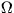, radians) PERIH D longitude or argument of perihelion (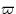or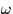, radians) AORQ D mean distance or perihelion distance (a or q, AU) E D eccentricity (e) AORL D mean anomaly or longitude (M or L, radians, JFORM=1,2 only) DM D daily motion (n, radians, JFORM=1 only)

RETURNED:

 D(13) U universal orbital elements (Note 1) (1) combined mass (M+m) (2) total energy of the orbit (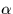) (3) reference (osculating) epoch (t0) (4-6) position at reference epoch (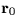) (7-9) velocity at reference epoch (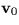) (10) heliocentric distance at reference epoch (11)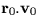(12) date (t) (13) universal eccentric anomaly (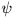) of date, approx JSTAT I status: 0 = OK -1 = illegal JFORM -2 = illegal E -3 = illegal AORQ -4 = illegal DM -5 = numerical error

NOTES:
1.
The universal'' elements are those which define the orbit for the purposes of the method of universal variables (see reference). They consist of the combined mass of the two bodies, an epoch, and the position and velocity vectors (arbitrary reference frame) at that epoch. The parameter set used here includes also various quantities that can, in fact, be derived from the other information. This approach is taken to avoiding unnecessary computation and loss of accuracy. The supplementary quantities are (i), which is proportional to the total energy of the orbit, (ii) the heliocentric distance at epoch, (iii) the outwards component of the velocity at the given epoch, (iv) an estimate of, the universal eccentric anomaly'' at a given date and (v) that date.
2.
The companion routine is sla_UE2PV. This takes the set of numbers that the present routine outputs and uses them to derive the object's position and velocity. A single prediction requires one call to the present routine followed by one call to sla_UE2PV; for convenience, the two calls are packaged as the routine sla_PLANEL. Multiple predictions may be made by again calling the present routine once, but then calling sla_UE2PV multiple times, which is faster than multiple calls to sla_PLANEL.
3.
DATE is the epoch of osculation. It is in the TT timescale (formerly Ephemeris Time, ET) and is a Modified Julian Date (JD-2400000.5).
4.
The supplied orbital elements are with respect to the J2000 ecliptic and equinox. The position and velocity parameters returned in the array U are with respect to the mean equator and equinox of epoch J2000, and are for the perihelion prior to the specified epoch.
5.
The universal elements returned in the array U are in canonical units (solar masses, AU and canonical days).
6.
Three different element-format options are supported, as follows.

JFORM=1, suitable for the major planets:


EPOCH 		 = 		 epoch of elements t0 (TT MJD)
ANODE 		 = 		 longitude of the ascending node(radians)
PERIH 		 = 		 longitude of perihelion(radians)
AORQ 		 = 		 mean distance a (AU)
E 		 = 		 eccentricity e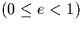AORL 		 = 		 mean longitude L (radians)
DM 		 = 		 daily motion n (radians)


JFORM=2, suitable for minor planets:


EPOCH 		 = 		 epoch of elements t0 (TT MJD)
ANODE 		 = 		 longitude of the ascending node(radians)
PERIH 		 = 		 argument of perihelion(radians)
AORQ 		 = 		 mean distance a (AU)
E 		 = 		 eccentricity eAORL 		 = 		 mean anomaly M (radians)


JFORM=3, suitable for comets:


EPOCH 		 = 		 epoch of perihelion T (TT MJD)
ANODE 		 = 		 longitude of the ascending node(radians)
PERIH 		 = 		 argument of perihelion(radians)
AORQ 		 = 		 perihelion distance q (AU)
E 		 = 		 eccentricity e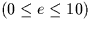7.
Unused elements (DM for JFORM=2, AORL and DM for JFORM=3) are not accessed.
8.
The algorithm was originally adapted from the EPHSLA program of D.H.P.Jones (private communication, 1996). The method is based on Stumpff's Universal Variables.

REFERENCE:
Everhart, E. & Pitkin, E.T., Am. J. Phys. 51, 712, 1983.Next: SLA_EPB - MJD to Besselian Epoch
Up: SUBPROGRAM SPECIFICATIONS
Previous: SLA_EG50 - B1950 to Galactic

SLALIB --- Positional Astronomy Library